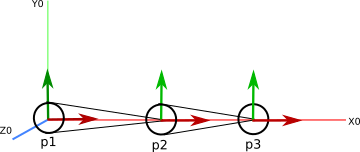## Name:

Due December 2, in lab

This worksheet is intended as a short 60 min exercise to be done in groups of 1-3 people.

In this question, you will work through the calculations needed to perform IK for a two-link chain on paper.

Remember that you can use python, octave, matlab, maple, our basecode or any other method to check your answers!

Suppose we have a 2-link chain as in class.• The root joint $p_1$ is located at the origin.

• The next joint $p_2$ is offset from $p_1$ by $(2,0,0)^T$

• The next joint $p_3$ is offset from $p_2$ by $(5,0,0)^T$

Reference: IK example

Suppose we wish to position $p_3$ at a target position $p_d = (-4,3,0)^T$

Let’s use the analytic IK method for class to compute rotations for $p_1$ and $p_2$ such that $p_3$ is located at $p_d$.

• What is the desired distance r between $p_3$ and $p_1$?

• What is L1?

• What is L2?

• What is the angle $\theta_{2z}$ that achieves the desired length?

• What is the new global position of joint 3? Verify that setting the rotation of joint 1 to $\theta_{2z}$ results in the desired distance.

### Use polar coordinates to compute the orientation of joint 1

• What is the angle $\theta_{1z}$ that points the limb along the x axis?

• What is the new global position of joint 3? Verify that setting $\theta_{2z}$ and $\theta_{1z}$ points the limb along the x axis using the kinematic equation for our joints.

• Compute the heading ($\beta$) and elevation ($\gamma$) that point the limb towards the target $p_d$.

• Plug in $\beta$, $\gamma$, $\theta_{1z}$, and $\theta_{2z}$ and verify that $p_3$ is now at location $p_d$.

### Find an angle/axis rotation to compute the orientation of joint 1

• After setting a rotation for joint2, what is the global position of joint 3?

• What is the direction vector $r$?

• What is the error vector $e$?

• What is the angle $\phi$ and axis of rotation?

• Plugin in the angle/axis rotation and $\theta_{2z}$ and verify that $p_3$ is now at location $p_d$